CE351 Lab 2020 Fall
The Arduino C Language
Name: Audra Benally
Email: albenally1@fortlewis.edu

1. Introduction

In this lecture, the fundamentals of the Arduino C language are introduced. The main topics in this lecture are data types, operators, the ‘for’ loop, the ‘while’ loop, the ‘if’ statement, and the ‘switch’ statement’. The following pictures and figures are the results of the Arduino C Programming Language Basics tutorial.

2. The Code and the Results

---------------------------- Task 1: Section 3 Example ----------------------------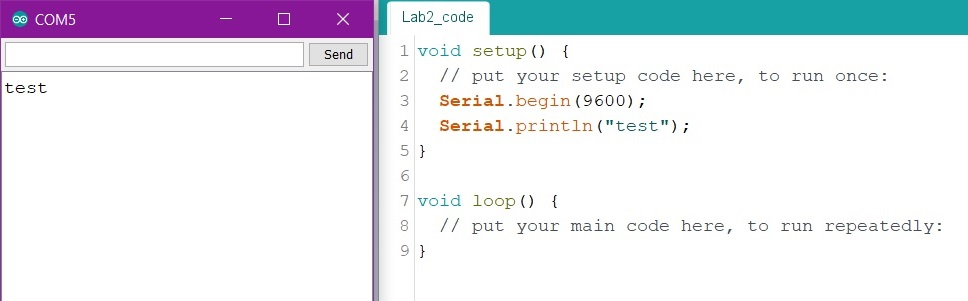Figure 1: Printing "test" to the serial monitor via the void setup() function.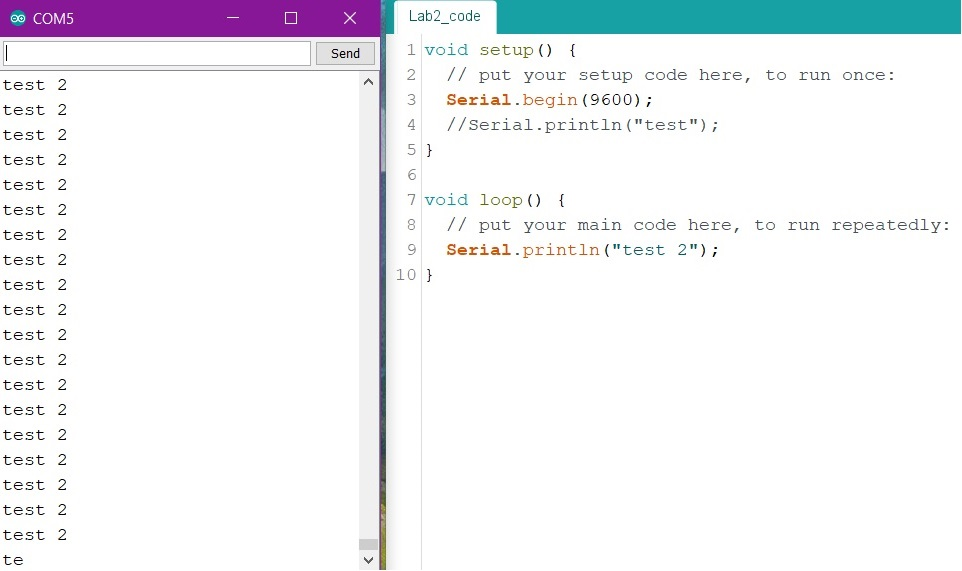Figure 2: Serial printing "test" via the void loop() function.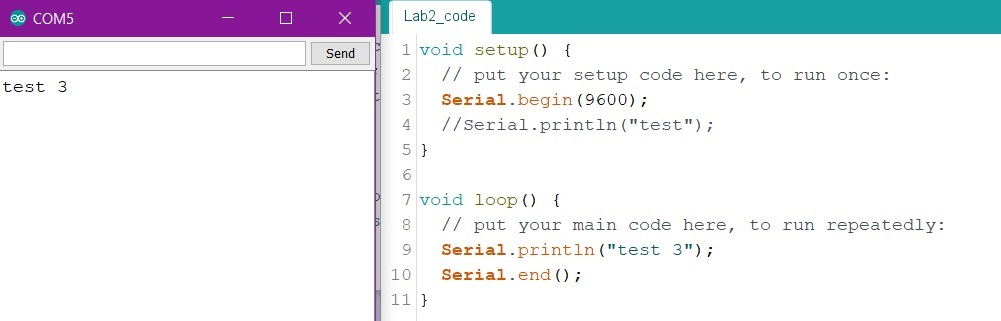Figure 3: Implementing the "Serial.end()" function in the void loop() section.

---------------------------- Task 1: Section 4 Example ----------------------------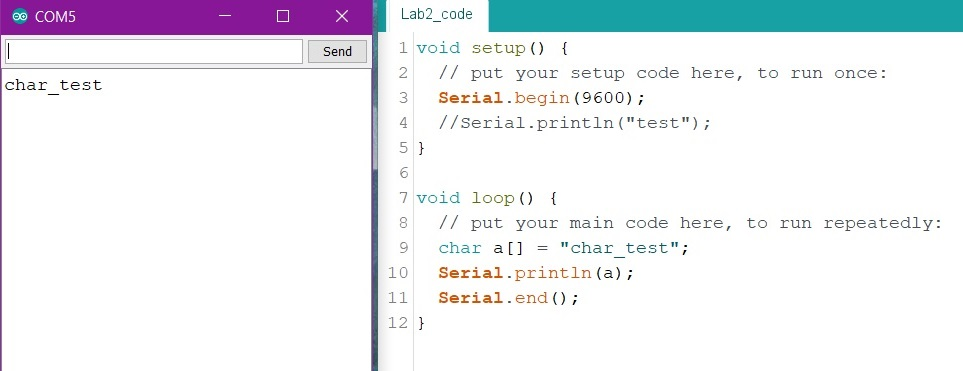Figure 4: Using a char array to hold values then printing the char variable.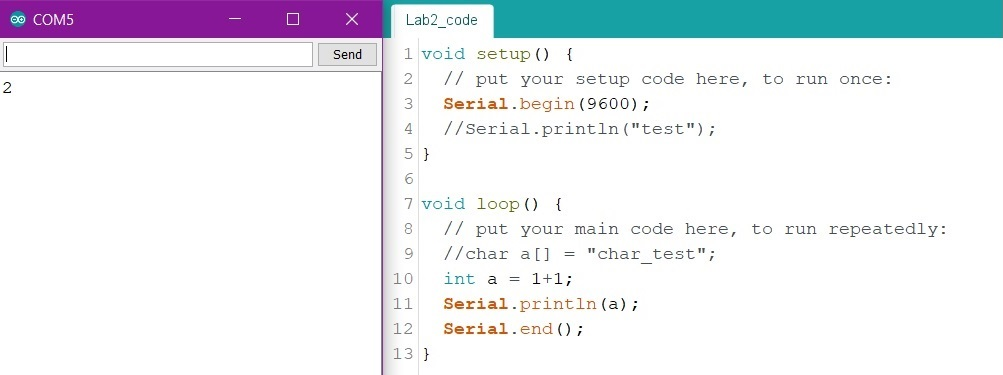Figure 5: Arduino arithmetic.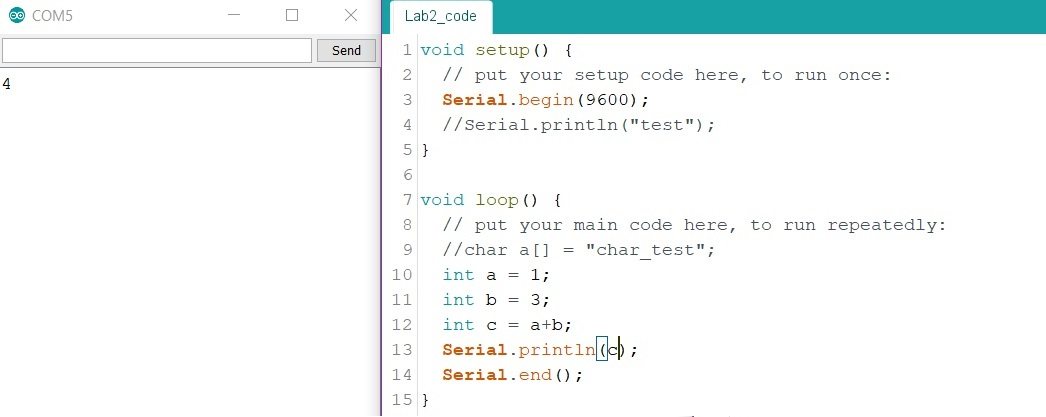Figure 6: More arduino math.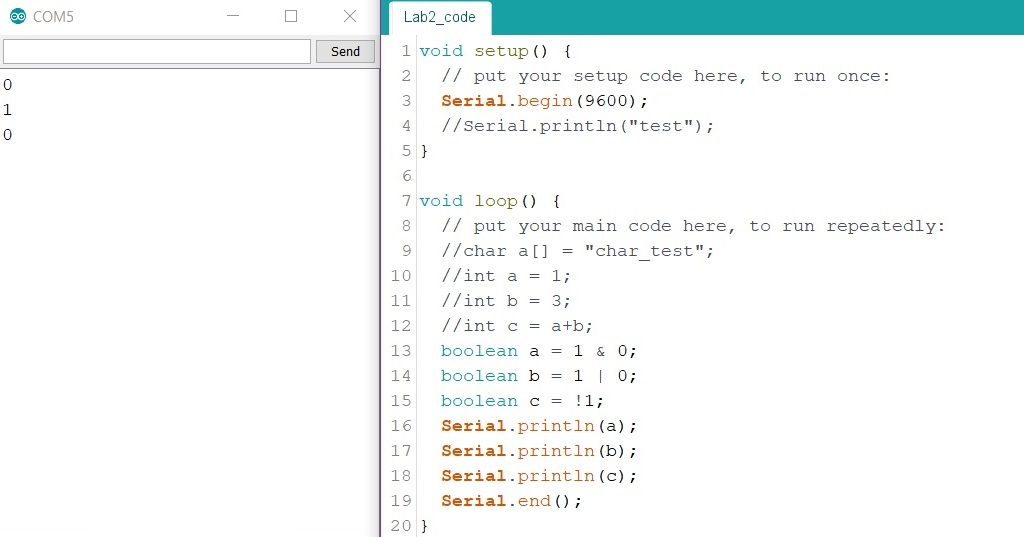Figure 7: Logic operations with boolean data types.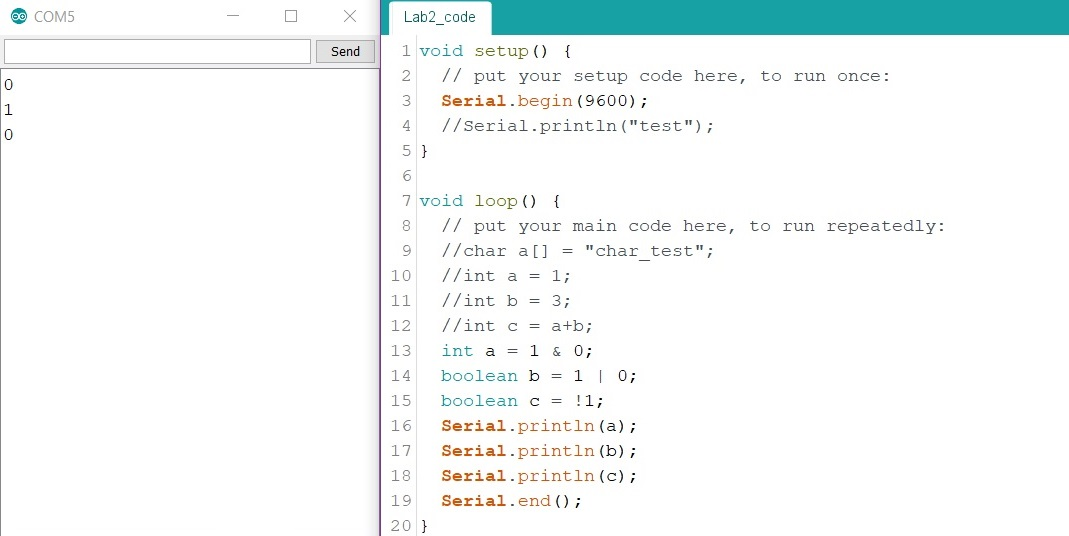Figure 8: Logic operators, exchanged one boolean for int data type.

---------------------------- Task 1: Section 5 Example ----------------------------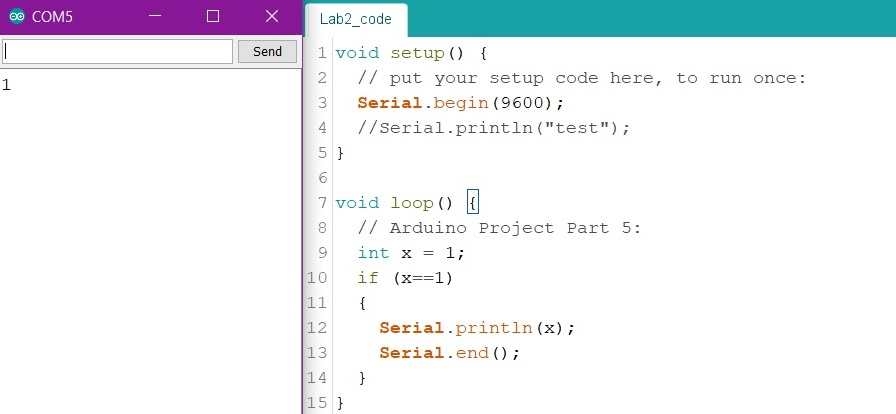Figure 9: The if statement. This process works by comparing two expressions. Here the x is compared to 1 (line 10), if x = 1 then the Serial.prinln(x) and Serial.end() statements activate. If x did not equal 1 then the statements would be skipped over.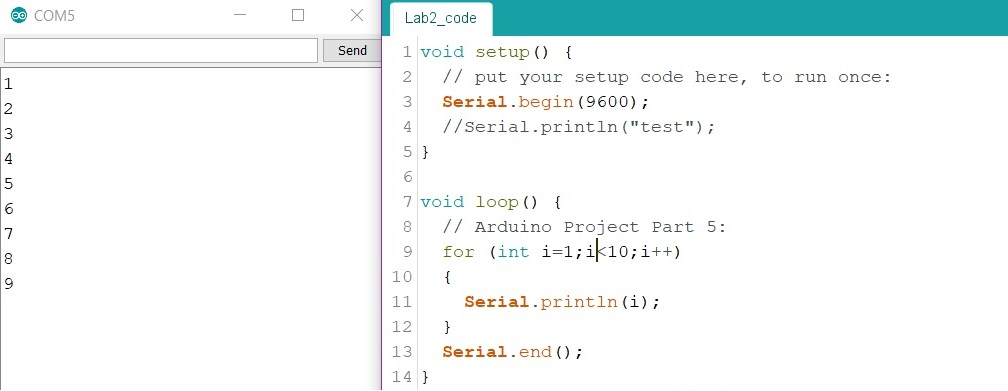Figure 10: The for loop. This portion of code loops through the code section in curly brackets a specific number of times depending on the expression directly following the "for". In this example, the for loops 9 times until i is equal to 10 then the looping stopps.Figure 11: The for loop combined with if statements. The for loopruns through the if statements during each iteration of the loop.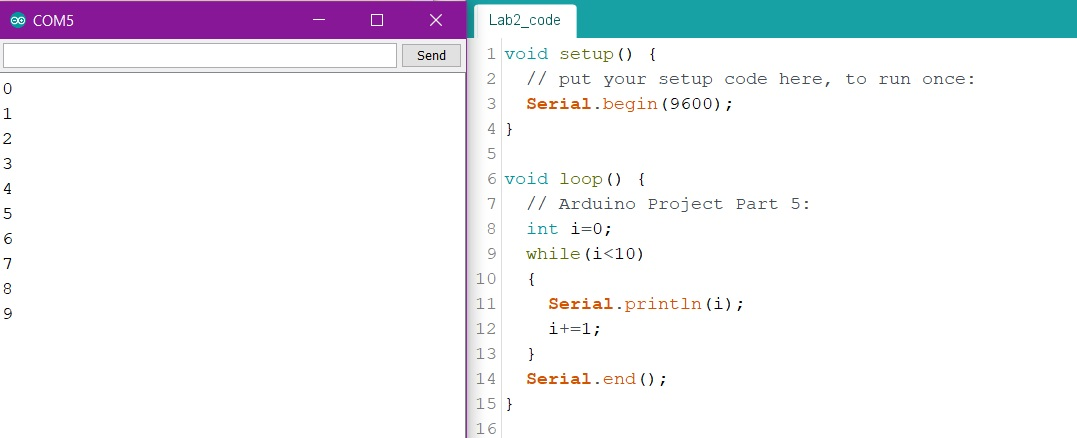Figure 12: The while loop. Similar to the for loop except the while loop will keep running through the specified code until a certain condition is met. Here, the loop will keep running as long as i < 10.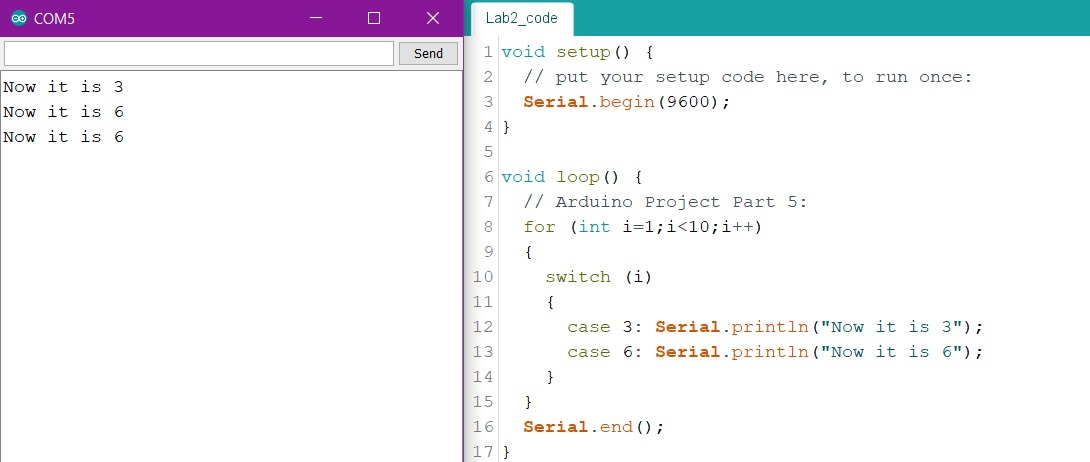Figure 13: The switch statement. Similar to the if statements, though the switch statement is more often used when there are a large number of options to choose from. Here, the switch statement is used without the "break;" lines that are needed after each case. If the break is missing then the switch statement may become faulty.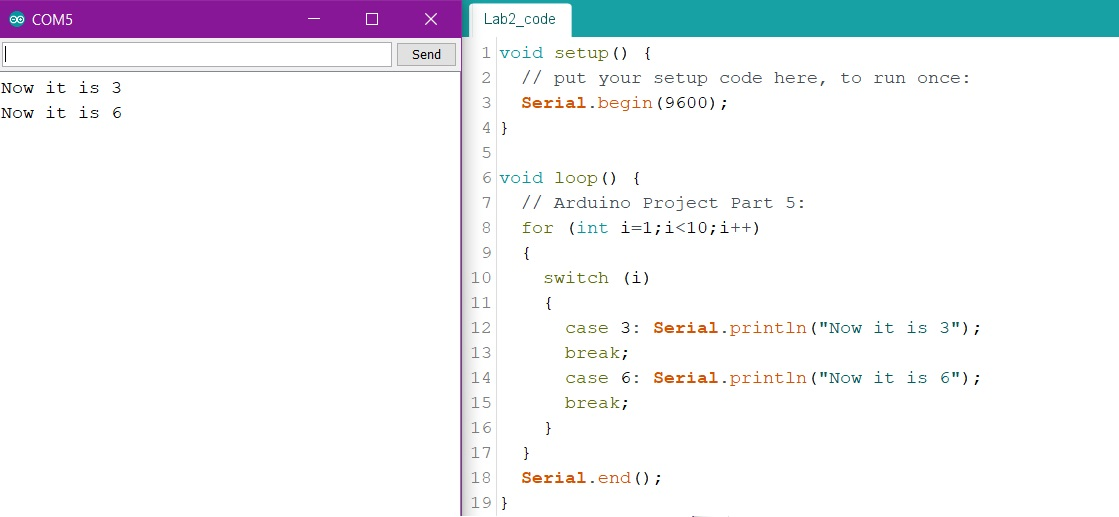Figure 14: The switch statement with the "break;" code lines after each case. Notice the different output from Figure 13.

---------------------------- Task 2 - do while loop ----------------------------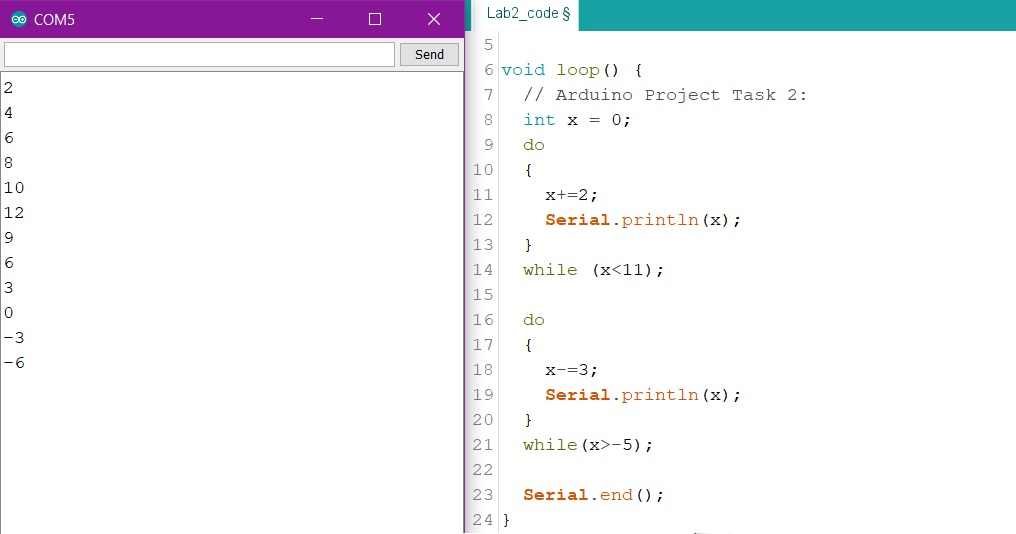Figure 15: The do while loop in two examples. The do while loop is almost exactly like the while loop except the order is different. The loop will always run at least once because the condition check takes place after the statements in curly brackets.

---------------------------- Task 3 - Hex Numbers ----------------------------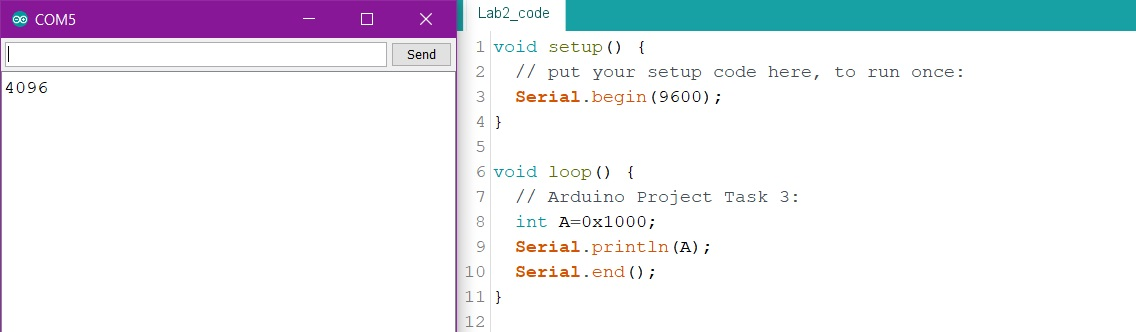Figure 16: 0x is used to represent Hex numbers. Here the int A variable is assigned the hex value 1000. The serial monitor displays the decimal value.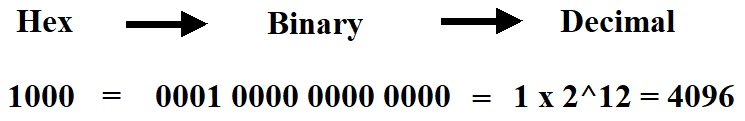Figure 17: The decimal value can be calculated by translating the hex value in to binary then in to decimal. The value of 0x1000 is the decimal value 4096. The value is verified in Figure 16.

---------------------------- Task 4 - More Hex Numbers ----------------------------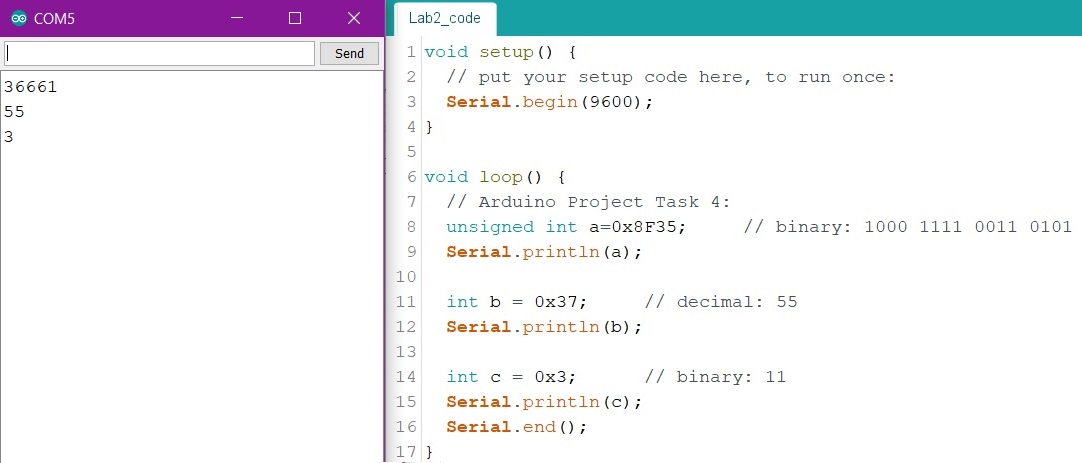Figure 18: The given numbers are converted into hex then assigned to variables corresponding to their question parts. The int data type is 16 bits. The unsigned int is needed for part a because the range of int does not reach 36661.

---------------------------- Task 5 - Hex Number Conversion ----------------------------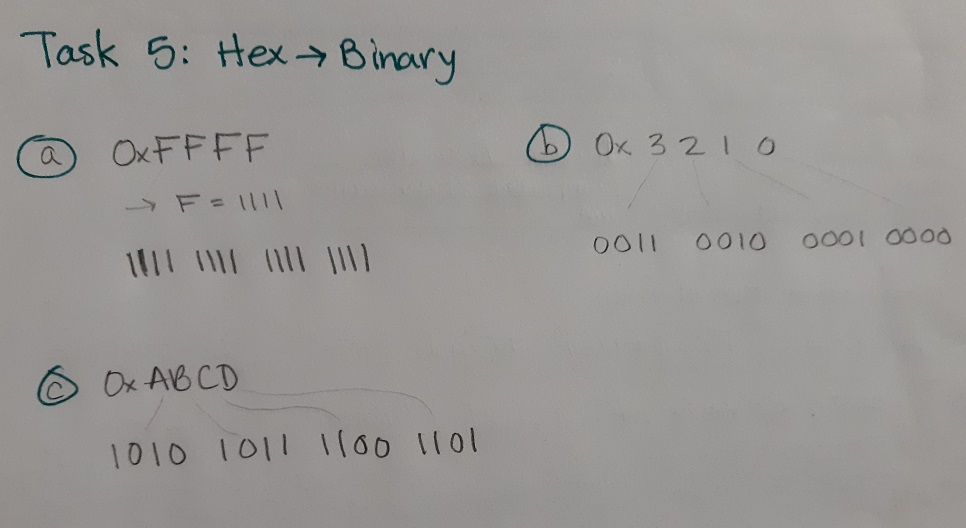Figure 19: The given hex numbers converted into binary. Here, each digit in hex just needs to be translated to the corresponding 4 bit binary number.

---------------------------- Task 6 - Binary Number Display Using Masks and LEDs ----------------------------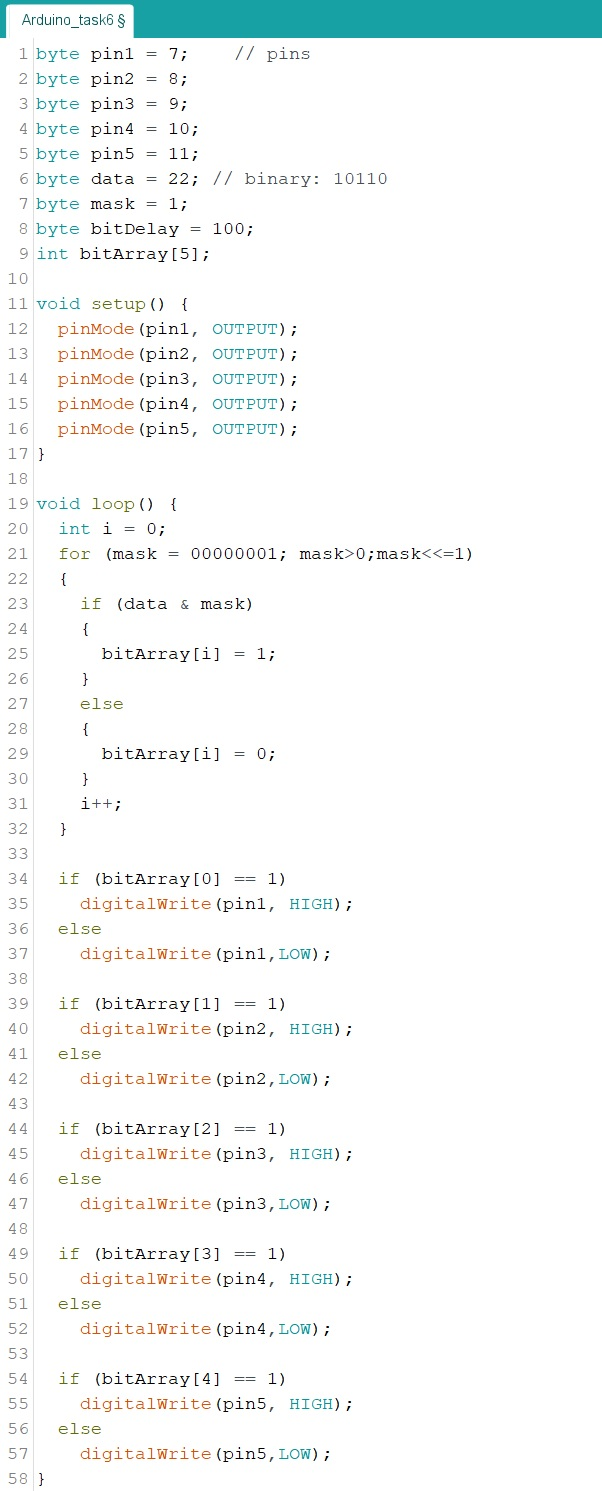Figure 20: Code to display a 5 bit binary number to a parallel 5 LED circuit system.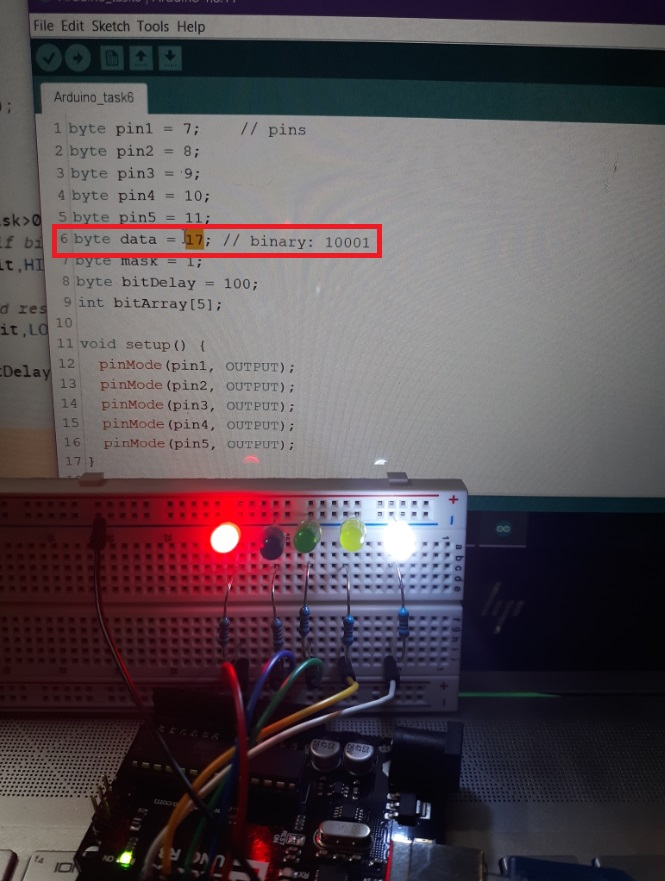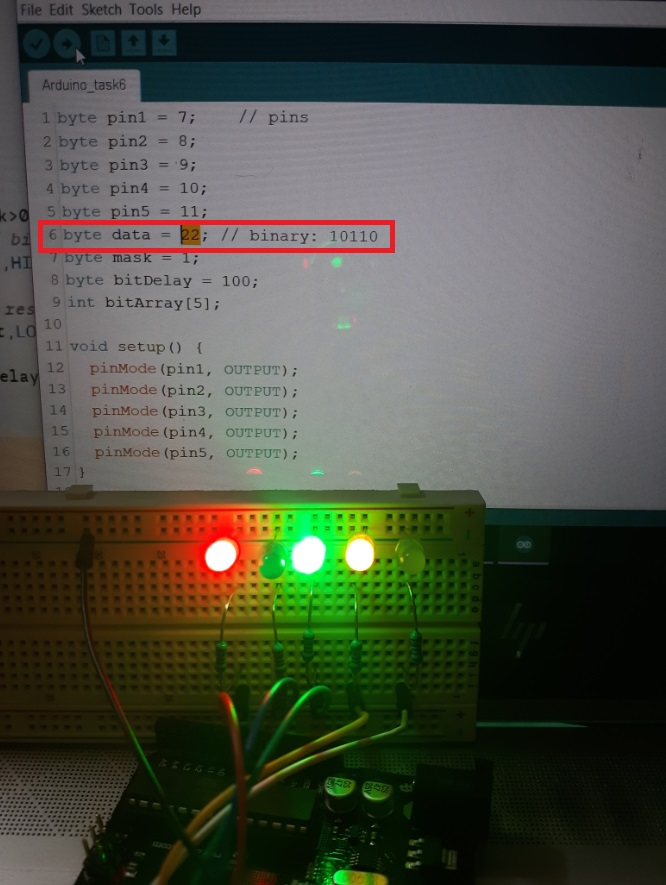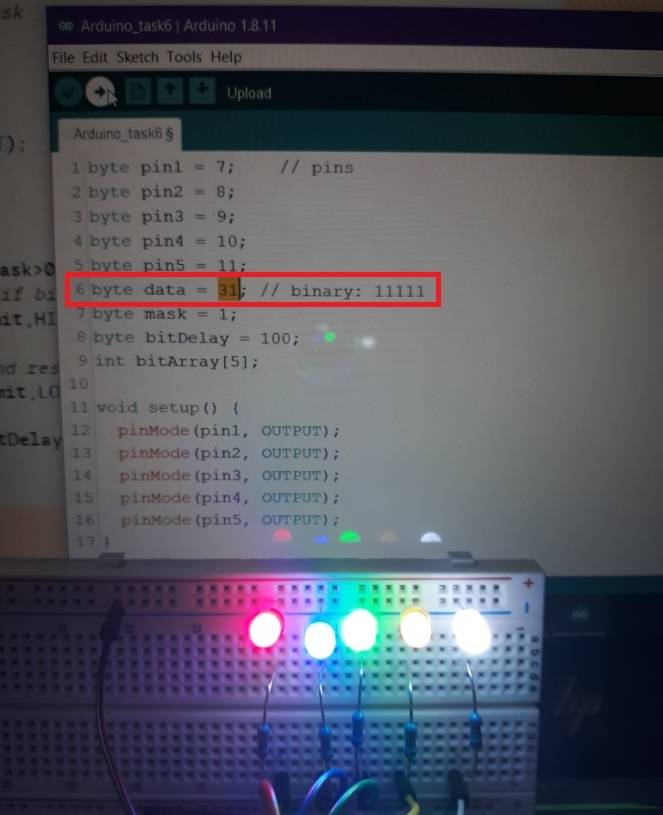Figure 21: A few examples of the Figure 20 code in action. The decimal and binary values can be seen in the red square on the computer screen.

The end (: